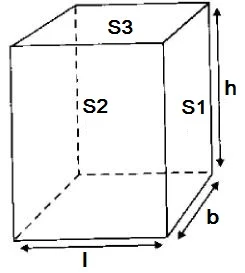#### To observe and compare the pressure exerted by a solid iron cuboid on fine sand/wheat flour while resting on it's three different faces and to calculate the pressure exerted in the three different cases - Science Practicals

Aim
To observe and compare the pressure exerted by a solid iron cuboid on fine sand/wheat flour while resting on it's three different faces and to calculate the pressure exerted in the three different cases.

Theory
• Force acting normally on a surface is called the thrust.
• The force (thrust) acting normally (perpendicularly) on the unit area of a surface is called pressure. If F is the force acting normally on a surface of area of cross-section A, then P = F/A.
• SI unit of pressure is Nm-2 which is also called pascal (Pa).
• Pressure is directly proportional to the force applied on a surface.
• Pressure is inversely proportional to the area of cross-section of a surface normal to the direction of force.

Materials Required
Spring balance, Cuboid, Sand, Tray to hold sand, Scale

ProcedureStep 1: The mass of the cuboid is found with the help of spring balance. Three observations are taken and the mean value is found. Calculated weight = mg (g = 9.8ms-2 )
Step 2: The length (l), breadth (b) and height (h) of the cuboid is found by taking three observations of each and then by  taking their mean.
Step 3: Three pairs of opposite surfaces are marked as S1, S2 and S3 as shown in the figure.
Step 4: The surface areas are calculated as S1 = lh, S2 = bh, S3 = lb
Step 5: The tray is placed on the horizontal surface, 2 inch thick layer of sand is put in it and the surface of the sand is flatten.
Step 6: The cuboid is gently placed vertically on the sand such that the surface S1 is on the sand.
Step 7: The cuboid is removed and the depth of the depression caused is found by using the scale and recorded. This is repeated two times after flattening the surface and the mean is taken.This mean is recorded as d1.
Step 8: The steps 6 and 7 are repeated so that surface S2 and S3 are on the surface of the loose sand. The depths of depression are recorded as d2 and d3.
Step 9: The pressures P1, P2 and P3 are calculated for the surfaces S1, S2 and S3 respectively by dividing the weight of the cuboid by the surface areas of contact.
Step 10: The calculated values of pressures and depths are compared.

Observations and Calculations
Range of the measuring scale =
Least count of the measuring scale =
Range of the spring balance =
Least count of the spring balance =
Length of the brick, 1 =  cm =
Breadth of the brick, b =  cm =
Height of the brick, h =  cm =
Mass of the brick, m =
Acceleration due to gravity at the place of experiment, g =
Weight of the brick = mg =
Force exerted by the brick when placed on the wet sand-bed in the tray F = mg =
Pressure of the brick on the wet sand-bed =

 Sl. No. Area of Face Pressure, P = F/A Weight of the dip, d 1. l×b = 2. b×h = 3. l×h =

Precautions
1. The sand should be leveled properly after every reading.
2. The block should be placed on the sand very gently, without applying any extra pressure.
3. The block has to be placed vertically.
4. The scale while measuring the depression should just touch the bottom of the depression.
5. The dimensions of the cuboid should be uniform.

Go to List of Experiments